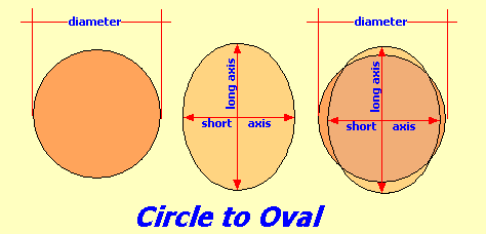# Changing a Circle to an Oval

This calculator converts the area of a circle into an oval area of the same size### Circle Formula's

R = D ÷ 2
where R = radius, D = diameter

Area;
A = π * D² ÷ 4
where A = area, π = 3.14159..., D = diameter

Circumference;
C = 2 * π * D ÷ 2
where C = circumference, π = 3.14159...., D = diameter

### Oval Formula's

Area
A = π * la * sa ; ÷ 4

Long Axis
La = (A / (π * (Sa ÷ 2)) * 2
where La = long axis, A = area, π = 3.14159...., Sa = short axis

Perimeter
S = Sa ÷ 2 , L = La ÷ 2
Pa = (S + L) * 3
Pb = ((3 * L + S) * (L + 3 * S))²
P = π * (Pa - Pb)

where P = perimeter, La = long axis, π = 3.14159...., Sa = short axis

### Circle to Oval

Select unit:
diameter of the circle {{selectedunit.m1}}
short axis of oval {{selectedunit.m1}}
decimal rounding # of digits in results

#### Results:

 circle's radius {{selectedunit.m1}} area of the circle {{selectedunit.m1}}² circumference of the circle {{selectedunit.m1}} long axis of the oval {{selectedunit.m1}} perimeter of the the oval {{selectedunit.m1}}

#### Calculation

1. enter the diameter of a circle
2. the length of the short axis of the oval
3. number of decimal places required in results

#### Results

2. the area of the circle
3. the perimeter of the circle (circumference)
4. the long axis length of the oval
5. perimeter of the oval